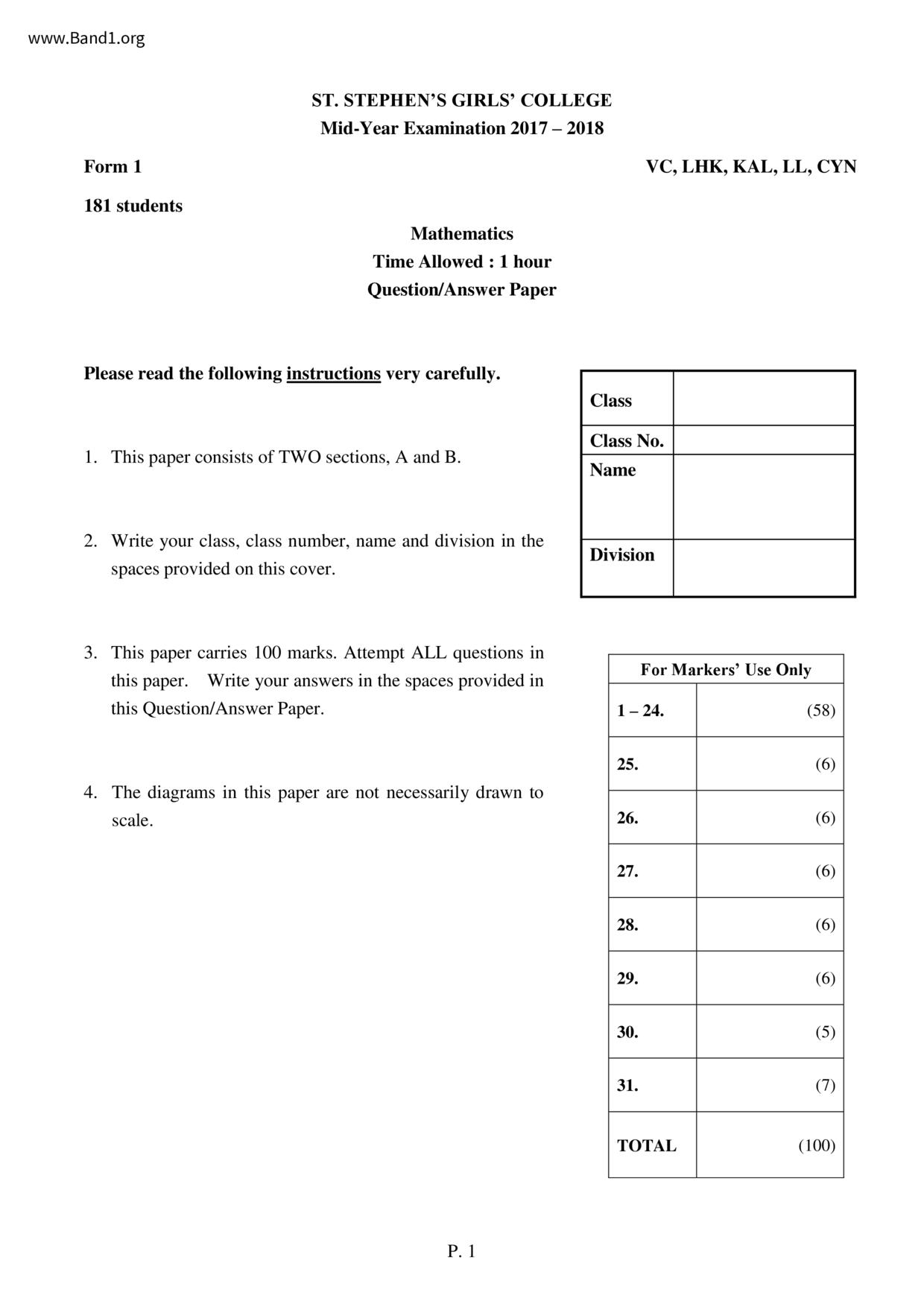# 中一 數學試卷 (F1 Maths Past Paper)

6929

pdf

10

st_stephen_girl_college_F1-Maths-17-18-my

▼ 圖片只作預覽, 如欲下載整份卷, 請按「免費成為會員」 ▼▲ 圖片只作預覽, 如欲下載整份卷, 請按「免費成為會員」 ▲

## 中一數學試卷 PDF 下載

181 students
ST. STEPHEN'S GIRLS' COLLEGE
Mid-Year Examination 2017 - 2018
Mathematics
Time Allowed : 1 hour
consists of TWO sections, A and B.
2. Write your class, class number, name and division in the
spaces provided on this cover.
3. This paper carries 100 marks. Attempt ALL questions in
4. The diagrams in this paper are not necessarily drawn to
VC, LHK, KAL, LL, CYN
For Markers' Use Only
Form 1 Mathematics Mid-Year Exam 2017-18
End of Paper
Form 1 Mathematics Mid-Year Exam 2017-18
Section A (58%)
All rough work should be done on the rough work paper provided, but will not be marked.
1. Divide +6 by the sum of +1 and -4. Find the result.
2. Arrange the following numbers in ascending order:
+3-1₁-3.5, -1, +4
3. Evaluate 6-(-1.5)+(+9)×|
4. Represent the following word phrase by an algebraic
expression:
Subtract the square of the sum of a and b from half of c.
5. For the algebraic expression 3xxy-12+20÷10×x,
(a) the number of terms,
(b) the constant term.
6. Solve the equation 20= 4(2-x).
7. Simplify 3a+4bxa-2a÷2+2ab.
8. The remaining stored value of John's octopus card is \$9.2.
After buying 6 bags of candy with this octopus card, the
remaining stored value becomes -\$23.8. Find the price of a
bag of candy.
9. Frank's weight is two-thirds of his father's weight. If their 9.
total weight is 120 kg, find the weight of Frank's father.
Form 1 Mathematics Mid-Year Exam 2017-18
10. Ben has 3 times as many sweets as Ken. After eating 14
sweets, Ben gives the remaining sweets to 4 friends. If
each friend gets 13 sweets, how many sweets does Ken
11. The sum of two consecutive integers is -435. Find the
larger integer.
12. Which of the following is a formula?
14. Set up an inequality to represent each of the following.
(a) The result of adding m to 8 is not greater than 20.
(b) The result of subtracting a from 4 squared is less than
13. The printing cost \$C of n pages of notes can be calculated 13.
by the formula C = 4 +0.25n. If n = 400, find the printing
15. Refer to the diagram. By observation only, name
(a) one acute angle;
(b) one obtuse angle.
16. Which of the following angles is the largest?
of a right angle
of a straight angle
of a round angle
of a round angle
Form 1 Mathematics Mid-Year Exam 2017-18
17. Classify the following triangle according to the sizes of its
18. If 45% of a number is 135, find the number.
19. There are altogether 36 red balls and white balls in a bag.
If 33% of the balls are white, find the number of red
balls in the bag.
20. In a school, there are 1200 students and 42% of the
students are boys. If 189 boys do not wear glasses, find the
percentage of boys who do not wear glasses.
21. Last year, the height of a plant was 40 cm. If the height of
the plant is 50 cm this year, find the percentage increase of
the height of the plant.
22. A dress is sold at a 24% discount and the selling price is
\$190. Find the marked price of the dress.
23. A shirt marked at \$150 is sold at a discount of 20%. If the
percentage profit is 60%, find the cost price of the shirt.
24. The weights of five tourists are given in the table below.
Paul Queenie
Weight 82 kg 53 kg
Rose Stanley Tom
76 kg 104 kg 99 kg
Estimate their total weight by rounding off each weight to
the nearest 10 kg.
Form 1 Mathematics Mid-Year Exam 2017-18
(42%) All working must be clearly shown in the spaces provided.
Find the product of the first two multiples of 5.
Find the sum of all the prime numbers between 10 and 18.
Using the results of (a) and (b), evaluate the following:
Subtract the product of the first two multiples of 5 from the sum of all the prime numbers
between 10 and 18.
26. It is given that a copper wire is no longer than 50 cm. It is then bent into a rectangle of length
x cm and width y cm.
Set up an inequality to represent the above situation.
Can the copper wire form a rectangle of length 16 cm and width 10 cm? Explain your
If the width of the rectangle formed is 11 cm, write down two possible lengths of the
rectangle formed.
Form 1 Mathematics Mid-Year Exam 2017-18
27. The price of a chair is \$10 more expensive than one-third of the price of a table. If John buys a
table and 4 chairs, he needs to pay \$250. Find the total price of a chair and a table. (6 marks)
Form 1 Mathematics Mid-Year Exam 2017-18
28. In the figure, PQR is a straight line. Find x and y.
Form 1 Mathematics Mid-Year Exam 2017-18
29. Solve the equation
Form 1 Mathematics Mid-Year Exam 2017-18
30. Alice weighs 45 kg. The weight of Betty is 10% higher than that of Alice.
(a) Find the weight of Betty.
(b) The weight of Alice is 10% lower than that of Christine. Do Betty and Christine have the